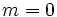# Prime power order implies subgroups of all orders dividing the group order

This article gives the statement and possibly, proof, of an implication relation between two group properties. That is, it states that every group satisfying the first group property (i.e., group of prime power order) must also satisfy the second group property (i.e., group having subgroups of all orders dividing the group order)
View all group property implications | View all group property non-implications
Get more facts about group of prime power order|Get more facts about group having subgroups of all orders dividing the group order

## Statement

Suppose$G$ is a group of prime power order$p^n$. Suppose$0 \le m \le n$. Then,$G$ has a subgroup of order$p^m$.

## Related facts

### Congruence condition on the number of subgroups

• Congruence condition on number of subgroups of given prime power order: This states that for any finite group$G$, and any prime power$p^m$ dividing the order of$G$, the number of subgroups of$G$ of order$p^m$ is congruent to$1$ modulo$p$. The special case of this (which is used as a first step in the proof) is where the order of$G$ is itself a power of$p$.

## Facts used

1. Prime power order implies not centerless

## Proof

Given: A group$G$ of prime power order$p^n$. An integer$m$ with$0 \le m \le n$.

To prove:$G$ has a subgroup of order$p^m$.

### Proof by moving upward on$m$

Proof: We prove the result by induction on$n$. The base case,$n = 0$, is trivial. Suppose$n \ge 1$. Note also that if$m = 0$, the trivial subgroup works, so we can consider$m \ge 1$.

1.$G$ has a normal subgroup$N$ of order$p$: Since$G$ is nontrivial, fact (1) tells us that its center$Z(G)$ is nontrivial. Pick any non-identity element$x \in Z(P)$. The order of$x$ is$p^j$, with <mah>1 \le j \le n[/itex]. Pick$y = x^{p^{j-1}}$.$N = \langle y \rangle$ has order$p$, and since$N \le Z(G)$,$N$ is normal.
2.$G/N$ has a subgroup of order$p^{m-1}$:$G/N$ has order$p^{n-1}$, so the induction hypothesis applies to yield that it has a subgroup, say$M/N$, of order$p^{m-1}$.
3. The subgroup$M$ has order$p^m$: This is a direct consequence of Lagrange's theorem, and what we know about the orders of$N$ and$M/N$.

### Proof by moving downward on$m$

Proof: This uses the idea that every maximal subgroup is normal, and has index$p$, hence we can find a subgroup of the required order inside the maximal subgroup. PLACEHOLDER FOR INFORMATION TO BE FILLED IN: [SHOW MORE]